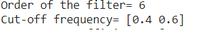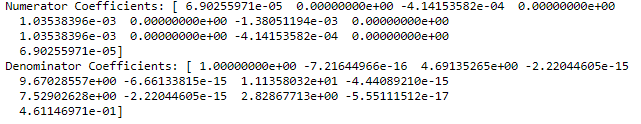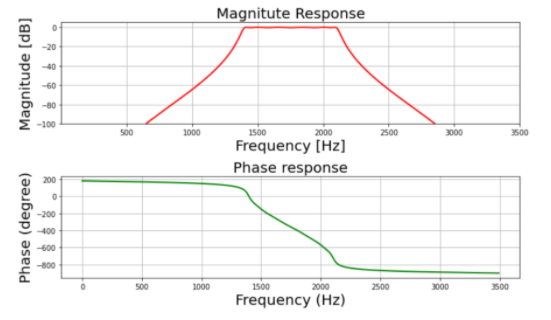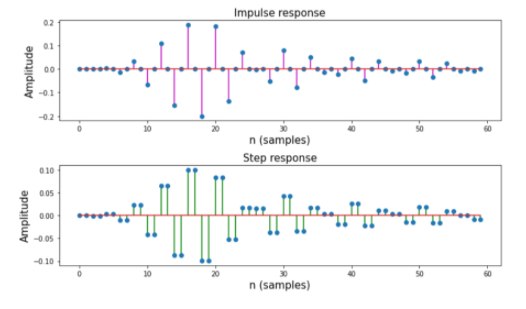# Design IIR Bandpass Chebyshev Type-1 Filter using Scipy – Python

• Last Updated : 10 Nov, 2021

IIR stands for Infinite Impulse Response, It is one of the striking features of many linear-time invariant systems that are distinguished by having an impulse response h(t)/h(n) which does not become zero after some point but instead continues infinitely.

## What is IIR Chebyshev Filter?

IIR Chebyshev is a filter that is linear-time invariant filter just like the Butterworth however, it has a steeper roll off compared to the Butterworth Filter. Chebyshev Filter is further classified as Chebyshev Type-I and Chebyshev Type-II according to the parameters such as pass band ripple and stop ripple.

## How is Chebyshev Filter different from Butterworth?

Chebyshev Filter has a steeper roll-off compared to the Butterworth Filter.

## What is Chebyshev Type-I Filter?

Chebyshev Type-I minimizes the absolute difference between the ideal and actual frequency response over the entire passband by incorporating an equal ripple in the passband.

The specifications are as follows:

• Pass band frequency: 1400-2100 Hz
• Stop band frequency: 1050-24500 Hz
• Pass band ripple: 0.4dB
• Stop band attenuation: 50 dB
• Sampling frequency: 7 kHz

We will plot the magnitude, phase, impulse, step response of the filter.

Step-by-step Approach:

Step 1: Importing all the necessary libraries.

## Python3

 `import` `numpy as np``import` `scipy.signal as signal``import` `matplotlib.pyplot as plt`

Step 2:Defining the user defined functions such as mfreqz() and impz().

## Python3

 `def` `mfreqz(b, a, Fs):``  ` `    ``# Compute frequency response of the filter``    ``# using signal.freqz function``    ``wz, hz ``=` `signal.freqz(b, a)` `    ``# Calculate Magnitude from hz in dB``    ``Mag ``=` `20``*``np.log10(``abs``(hz))` `    ``# Calculate phase angle in degree from hz``    ``Phase ``=` `np.unwrap(np.arctan2(np.imag(hz), np.real(hz)))``*``(``180``/``np.pi)``    ` `    ``# Calculate frequency in Hz from wz``    ``Freq ``=` `wz``*``Fs``/``(``2``*``np.pi)``    ` `    ``# Plot filter magnitude and phase responses using subplot.``    ``fig ``=` `plt.figure(figsize``=``(``10``, ``6``))` `    ``# Plot Magnitude response``    ``sub1 ``=` `plt.subplot(``2``, ``1``, ``1``)``    ``sub1.plot(Freq, Mag, ``'r'``, linewidth``=``2``)``    ``sub1.axis([``1``, Fs``/``2``, ``-``100``, ``5``])``    ``sub1.set_title(``'Magnitude Response'``, fontsize``=``20``)``    ``sub1.set_xlabel(``'Frequency [Hz]'``, fontsize``=``20``)``    ``sub1.set_ylabel(``'Magnitude [dB]'``, fontsize``=``20``)``    ``sub1.grid()` `    ``# Plot phase angle``    ``sub2 ``=` `plt.subplot(``2``, ``1``, ``2``)``    ``sub2.plot(Freq, Phase, ``'g'``, linewidth``=``2``)``    ``sub2.set_ylabel(``'Phase (degree)'``, fontsize``=``20``)``    ``sub2.set_xlabel(r``'Frequency (Hz)'``, fontsize``=``20``)``    ``sub2.set_title(r``'Phase response'``, fontsize``=``20``)``    ``sub2.grid()` `    ``plt.subplots_adjust(hspace``=``0.5``)``    ``fig.tight_layout()``    ``plt.show()` `# Define impz(b,a) to calculate impulse response``# and step response of a system``# input: b= an array containing numerator coefficients,``#a= an array containing denominator coefficients``def` `impz(b, a):``  ` `    ``# Define the impulse sequence of length 60``    ``impulse ``=` `np.repeat(``0.``, ``60``)``    ``impulse[``0``] ``=` `1.``    ``x ``=` `np.arange(``0``, ``60``)` `    ``# Compute the impulse response``    ``response ``=` `signal.lfilter(b, a, impulse)` `    ``# Plot filter impulse and step response:``    ``fig ``=` `plt.figure(figsize``=``(``10``, ``6``))``    ``plt.subplot(``211``)``    ``plt.stem(x, response, ``'m'``, use_line_collection``=``True``)``    ``plt.ylabel(``'Amplitude'``, fontsize``=``15``)``    ``plt.xlabel(r``'n (samples)'``, fontsize``=``15``)``    ``plt.title(r``'Impulse response'``, fontsize``=``15``)` `    ``plt.subplot(``212``)``    ``step ``=` `np.cumsum(response)``    ` `    ``# Compute step response of the system``    ``plt.stem(x, step, ``'g'``, use_line_collection``=``True``)``    ``plt.ylabel(``'Amplitude'``, fontsize``=``15``)``    ``plt.xlabel(r``'n (samples)'``, fontsize``=``15``)``    ``plt.title(r``'Step response'``, fontsize``=``15``)``    ``plt.subplots_adjust(hspace``=``0.5``)` `    ``fig.tight_layout()``    ``plt.show()`

Step 3:Define variables with the given specifications of the filter.

## Python3

 `# Given specification``Fs ``=` `7000`  `# Sampling frequency in Hz``fp ``=` `np.array([``1400``, ``2100``])  ``# Pass band frequency in Hz``fs ``=` `np.array([``1050``, ``2450``])  ``# Stop band frequency in Hz``Ap ``=` `0.4`  `# Pass band ripple in dB``As ``=` `50`  `# stop band attenuation in dB`

Step 4: Computing the cut-off frequency

## Python3

 `# Compute pass band and stop band edge frequencies``wp ``=` `fp``/``(Fs``/``2``)  ``# Normalized passband edge frequencies w.r.t. Nyquist rate``ws ``=` `fs``/``(Fs``/``2``)  ``# Normalized stopband edge frequencies`

Step 5: Compute cut-off frequency & order

## Python3

 `# Compute order of the  Chebyshev type-1 filter using signal.cheb1ord``N, wc ``=` `signal.cheb1ord(wp, ws, Ap, As)` `# Print the order of the filter and cutoff frequencies``print``(``'Order of the filter='``, N)``print``(``'Cut-off frequency='``, wc)`

Output:Step 6: Compute the filter co-efficient

## Python

 `# Design digital Chebyshev type-1 filter``# using signal.cheby1 function``z, p ``=` `signal.cheby1(N, Ap, wc, ``'bandpass'``)` `# Print numerator and denomerator coefficients``# of the filter``print``(``'Numerator Coefficients:'``, z)``print``(``'Denominator Coefficients:'``, p)`

Output:Step 7: Plotting the Magnitude & Phase Response

## Python3

 `# Call mfreqz to plot the magnitude and phase response``mfreqz(z, p, Fs)`

Output:Step 8: Plotting the Impulse and Step Response

## Python3

 `# Call impz function to plot impulse``# and step response of the filter``impz(z,p)`

Output:Full Code:

## Python3

 `# import required library``import` `numpy as np``import` `scipy.signal as signal``import` `matplotlib.pyplot as plt`  `def` `mfreqz(b, a, Fs):``  ` `    ``# Compute frequency response of the filter``    ``# using signal.freqz function``    ``wz, hz ``=` `signal.freqz(b, a)` `    ``# Calculate Magnitude from hz in dB``    ``Mag ``=` `20``*``np.log10(``abs``(hz))` `    ``# Calculate phase angle in degree from hz``    ``Phase ``=` `np.unwrap(np.arctan2(np.imag(hz), np.real(hz)))``*``(``180``/``np.pi)``    ` `    ``# Calculate frequency in Hz from wz``    ``Freq ``=` `wz``*``Fs``/``(``2``*``np.pi)``    ` `    ``# Plot filter magnitude and phase responses using subplot.``    ``fig ``=` `plt.figure(figsize``=``(``10``, ``6``))` `    ``# Plot Magnitude response``    ``sub1 ``=` `plt.subplot(``2``, ``1``, ``1``)``    ``sub1.plot(Freq, Mag, ``'r'``, linewidth``=``2``)``    ``sub1.axis([``1``, Fs``/``2``, ``-``100``, ``5``])``    ``sub1.set_title(``'Magnitude Response'``, fontsize``=``20``)``    ``sub1.set_xlabel(``'Frequency [Hz]'``, fontsize``=``20``)``    ``sub1.set_ylabel(``'Magnitude [dB]'``, fontsize``=``20``)``    ``sub1.grid()` `    ``# Plot phase angle``    ``sub2 ``=` `plt.subplot(``2``, ``1``, ``2``)``    ``sub2.plot(Freq, Phase, ``'g'``, linewidth``=``2``)``    ``sub2.set_ylabel(``'Phase (degree)'``, fontsize``=``20``)``    ``sub2.set_xlabel(r``'Frequency (Hz)'``, fontsize``=``20``)``    ``sub2.set_title(r``'Phase response'``, fontsize``=``20``)``    ``sub2.grid()` `    ``plt.subplots_adjust(hspace``=``0.5``)``    ``fig.tight_layout()``    ``plt.show()` `# Define impz(b,a) to calculate impulse response``# and step response of a system``# input: b= an array containing numerator coefficients,``# a= an array containing denominator coefficients``def` `impz(b, a):``  ` `    ``# Define the impulse sequence of length 60``    ``impulse ``=` `np.repeat(``0.``, ``60``)``    ``impulse[``0``] ``=` `1.``    ``x ``=` `np.arange(``0``, ``60``)` `    ``# Compute the impulse response``    ``response ``=` `signal.lfilter(b, a, impulse)` `    ``# Plot filter impulse and step response:``    ``fig ``=` `plt.figure(figsize``=``(``10``, ``6``))``    ``plt.subplot(``211``)``    ``plt.stem(x, response, ``'m'``, use_line_collection``=``True``)``    ``plt.ylabel(``'Amplitude'``, fontsize``=``15``)``    ``plt.xlabel(r``'n (samples)'``, fontsize``=``15``)``    ``plt.title(r``'Impulse response'``, fontsize``=``15``)` `    ``plt.subplot(``212``)``    ``step ``=` `np.cumsum(response)  ``# Compute step response of the system``    ``plt.stem(x, step, ``'g'``, use_line_collection``=``True``)``    ``plt.ylabel(``'Amplitude'``, fontsize``=``15``)``    ``plt.xlabel(r``'n (samples)'``, fontsize``=``15``)``    ``plt.title(r``'Step response'``, fontsize``=``15``)``    ``plt.subplots_adjust(hspace``=``0.5``)` `    ``fig.tight_layout()``    ``plt.show()`  `# Given specification``Fs ``=` `7000`  `# Sampling frequency in Hz``fp ``=` `np.array([``1400``, ``2100``])  ``# Pass band frequency in Hz``fs ``=` `np.array([``1050``, ``2450``])  ``# Stop band frequency in Hz``Ap ``=` `0.4`  `# Pass band ripple in dB``As ``=` `50`  `# stop band attenuation in dB`  `# Compute pass band and stop band edge frequencies``wp ``=` `fp``/``(Fs``/``2``)  ``# Normalized passband edge frequencies w.r.t. Nyquist rate``ws ``=` `fs``/``(Fs``/``2``)  ``# Normalized stopband edge frequencies` `# Compute order of the  Chebyshev type-1``# filter using signal.cheb1ord``N, wc ``=` `signal.cheb1ord(wp, ws, Ap, As)` `# Print the order of the filter and cutoff frequencies``print``(``'Order of the filter='``, N)``print``(``'Cut-off frequency='``, wc)` `# Design digital Chebyshev type-1 filter using``# signal.cheby1 function``z, p ``=` `signal.cheby1(N, Ap, wc, ``'bandpass'``)`  `# Print numerator and denomerator coefficients of the filter``print``(``'Numerator Coefficients:'``, z)``print``(``'Denominator Coefficients:'``, p)` `# Call mfreqz to plot the magnitude and phase response``mfreqz(z, p, Fs)` `# Call impz function to plot impulse and``# step response of the filter``impz(z, p)`

Output:My Personal Notes arrow_drop_up